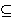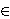### Irena Kosi-Ulbl and Joso Vukman

Faculty of Mechanical Engineering, University of Maribor, Smetanova ul. 17, Maribor, Slovenia
e-mail: irena.kosi@uni-mb.si

Department of Mathematics and Computer Science, FNM, University of Maribor, Koroska 160, Maribor, Slovenia
e-mail: joso.vukman@uni-mb.si

Abstract.   The main purpose of this paper is to prove the following result. Let X be a real or complex Banach space, let L(X) be the algebra of all bounded linear operators on X, let A(X)L(X) be a standard operator algebra, and let T : A(X) → L(X) be an additive mapping satisfying the relation T(A2n+1) = ∑i=12n+1 (-1)i+1 Ai-1 T(A) A2n+1-i, for all AA(X) and some fixed integer n ≥ 1. In this case T\ is of the form T(A) = AB + BA, for all AA(X) and some fixed BL(X). In particular, T is continuous.

2000 Mathematics Subject Classification.   46K15, 39B05.

Key words and phrases.   Prime ring, semiprime ring, Banach space, standard operator algebra.

Full text (PDF) (free access)

DOI: 10.3336/gm.44.2.11

References:

1. K. I. Beidar, W. S. Martindale III and A.V. Mikhalev, Rings with generalized identities, Marcel Dekker, Inc. New York, 1996.
MathSciNet

2. K. I. Beidar, M. Bresar, M.A. Chebotar and W. S. Martindale 3 rd, On Herstein's Lie map conjectures II, J. Algebra 238 (2001), 239-264.
MathSciNet     CrossRef

3. P. R. Chernoff, Representations, automorphisms, and derivations of some operator algebras, J. Functional Analysis 12 (1973), 275-289.
MathSciNet     CrossRef

4. J. Cusack, Jordan derivations on rings, Proc. Amer. Math. Soc. 53 (1975), 321-324.
MathSciNet     CrossRef

5. M. Fosner and D. Ilisevic, On a class of projections on *-rings, Commun. Algebra 33 (2005), 3293-3310.
MathSciNet     CrossRef

6. M. Fosner and J. Vukman, On some equations in prime rings, Monatsh. Math. 152 (2007), 135-150.
MathSciNet     CrossRef

7. I. N. Herstein, Jordan derivations of prime rings, Proc. Amer. Math. Soc. 8 (1957), 1104-1110.
MathSciNet     CrossRef

8. G. N. Jacobson, Structure of rings, American Mathematical Society, New York, 1956.
MathSciNet

9. I. Kosi-Ulbl and J. Vukman, An identity related to derivations of standard operator algebras and semisimple H*-algebras, CUBO A Math. J., to appear.

10. L. L. Stachó and B. Zalar, Bicircular projections on some matrix and operator spaces, Linear Algebra Appl. 384 (2004), 9-20.
MathSciNet     CrossRef

11. L. L. Stachó and B. Zalar, Bicircular projections and characterization of Hilbert spaces, Proc. Amer. Math. Soc. 132 (2004), 3019-3025.
MathSciNet     CrossRef

12. P. Semrl, Ring derivations on standard operator algebras, J. Funct. Anal. 112 (1993), 318-324.
MathSciNet     CrossRef

13. J. Vukman, On automorphisms and derivations of operator algebras, Glasnik Mat. Ser. III 19(39) (1984), 135-138.
MathSciNet

14. J. Vukman, I. Kosi-Ulbl and D. Eremita, On certain equations in rings, Bull. Austral. Math. Soc. 71 (2005), 53-60.
MathSciNet     CrossRef

15. J. Vukman, On functional equations related to bicircular projections, Glasnik Mat. Ser. III 41(61) (2006), 51-55.
MathSciNet     CrossRef

16. J. Vukman, On derivations of standard operator algebras and semisimple H*-algebras, Studia Sci. Math. Hungar. 44 (2007), 57-63.
MathSciNet     CrossRef